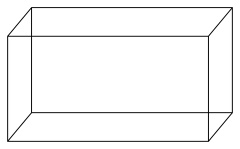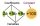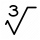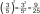# Prism X

The prism with the edges of the lengths x cm, 2x cm and 3x cm has volume 20250 cm3. What is the area of surface of the prism?

Correct result:

S =  4950 cm2

#### Solution:Our examples were largely sent or created by pupils and students themselves. Therefore, we would be pleased if you could send us any errors you found, spelling mistakes, or rephasing the example. Thank you!

Please write to us with your comment on the math problem or ask something. Thank you for helping each other - students, teachers, parents, and problem authors.Savage Math Hacker 21
i think that all math is great but cubes graphic is better.????????Dr Math
graphics fixedTips to related online calculators
Do you want to convert length units?

#### You need to know the following knowledge to solve this word math problem:

We encourage you to watch this tutorial video on this math problem:

## Next similar math problems:

• Alien shipThe alien ship has the shape of a sphere with a radius of r = 3000m, and its crew needs the ship to carry the collected research material in a cuboid box with a square base. Determine the length of the base and (and height h) so that the box has the large
• EightEight small Christmas balls with a radius of 1 cm have the same volume as one large Christmas ball. What has a bigger surface: eight small balls, or one big ball?
• Cuboid to cubeA cuboid with dimensions of 9 cm, 6 cm, and 4 cm has the same volume as a cube. Calculate the surface of this cube.
• The half lifeThe half-life of a radioactive isotope is the time it takes for a quantity of the isotope to be reduced to half its initial mass. Starting with 145 grams of a radioactive isotope, how much will be left after 3 half-lives?
• Length of the edgeFind the length of the edge of a cube that has a cm2 surface and a volume in cm3 expressed by the same number.
• An exampleAn example is playfully for grade 6 from Math and I don't know how to explain it to my daughter when I don't want to use the calculator to calculate the cube root. Thus: A cuboid was made from a block of 16x18x48 mm of modeline. What will be the edge of t
• Magnified cubeIf the lengths of the edges of the cube are extended by 5 cm, its volume will increase by 485 cm3. Determine the surface of both the original and the magnified cube.
• Cuboid and ratioFind the dimensions of a cuboid having a volume of 810 cm3 if the lengths of its edges coming from the same vertex are in ratio 2: 3: 5
• Wall thicknessThe hollow metal ball has an outside diameter of 40 cm. Determine the wall thickness if the weight is 25 kg and the metal density is 8.45 g/cm3.
• The ballThe ball has a radius of 2m. What percentage of the surface and volume is another sphere whose radius is 20% larger?
• Cube surfce2volumeCalculate the volume of the cube if its surface is 150 cm2.
• Right circular coneThe volume of a right circular cone is 5 liters. Calculate the volume of the two parts into which the cone is divided by a plane parallel to the base, one-third of the way down from the vertex to the base.
• Spherical segmentCalculate the volume of a spherical segment 18 cm high. The diameter of the lower base is 80 cm, the upper base 60 cm.
• Simplest formFind the simplest form of the following expression: 3 to the 2nd power - 1/4 to the 2nd power
• One third powerWhich equation justifies why ten to the one-third power equals the cube root of ten?
• Divide 8Divide 6840 by x y and z, in such a way that x has twice as much as y, who has half as much as z
• Three members GPThe sum of three numbers in GP (geometric progression) is 21 and the sum of their squares is 189. Find the numbers.